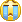# 强化学习系列之一:马尔科夫决策过程

机器学习一共有三个分支，有监督学习、无监督学习和强化学习。强化学习是系统从环境学习以使得奖励最大的机器学习。强化学习和有监督学习的不同在于教师信号。强化学习的教师信号是动作的奖励，有监督学习的教师信号是正确的动作。

我最近在学习强化学习。为了整理学习心得，开始写这个强化学习系列博客。由于学识浅薄，内容难免有些错误，还望各路大神不吝赐教。

### 1. 马尔科夫决策过程

要说强化学习，就必须说说马尔科夫决策过程 (Markov Decision Processes, MDP)。马尔可夫决策过程是基于马尔可夫过程理论的随机动态系统的决策过程，其分五个部分：

1. $$S$$ 表示状态集 (states)；
2. $$A$$ 表示动作集 (Action)；
3. $$P_{s,a}^{s’}$$ 表示状态 s 下采取动作 a 之后转移到 s’ 状态的概率；
4. $$R_{s,a}$$ 表示状态 s 下采取动作 a 获得的奖励；
5. $$\gamma$$ 是衰减因子。

和一般的马尔科夫过程不同，马尔科夫决策过程考虑了动作，即系统下个状态不仅和当前的状态有关，也和当前采取的动作有关。还是举下棋的例子，当我们在某个局面（状态s）走了一步 (动作 a )。这时对手的选择（导致下个状态 s’ ）我们是不能确定的，但是他的选择只和 s 和 a 有关，而不用考虑更早之前的状态和动作，即 s’ 是根据 s 和 a 随机生成的。

下图是一个机器人从任意一个状态出发寻找金币的例子。找到金币则获得奖励 1，碰到海盗则损失 1。找到金币或者碰到海盗则机器人停止。

class Mdp:

def __init__(self):

self.states         = [1,2,3,4,5,6,7,8] # 0 indicates end
self.terminal_states      = dict()
self.terminal_states   = 1
self.terminal_states   = 1
self.terminal_states   = 1

self.actions        = ['n','e','s','w']

self.rewards        = dict();
self.rewards['1_s'] = -1.0
self.rewards['3_s'] = 1.0
self.rewards['5_s'] = -1.0

self.t              = dict();
self.t['1_s']       = 6
self.t['1_e']       = 2
self.t['2_w']       = 1
self.t['2_e']       = 3
self.t['3_s']       = 7
self.t['3_w']       = 2
self.t['3_e']       = 4
self.t['4_w']       = 3
self.t['4_e']       = 5
self.t['5_s']       = 8
self.t['5_w']       = 4

self.gamma          = 0.8

def transform(self, state, action): ##return is_terminal,state, reward
if state in self.terminal_states:
return True, state, 0

key = '%d_%s'%(state, action);
if key in self.t:
next_state = self.t[key];
else:
next_state = state

is_terminal = False
if next_state in self.terminal_states:
is_terminal = True

if key not in self.rewards:
r = 0.0
else:
r = self.rewards[key];

return is_terminal, next_state, r;


马尔科夫决策过程是强化学习的理论基础。不管我们是将强化学习应用于五子棋游戏、星际争霸还是机器人行走，我们都假设背后存在了一个马尔科夫决策过程。只不过有的时候我们知道马尔科夫决策过程所有信息(状态集合，动作集合，转移概率和奖励)，有的时候我们只知道部分信息 (状态集合和动作集合)，还有些时候马尔科夫决策过程的信息太大无法全部存储 (比如围棋的状态集合总数为$$3^{19\times 19}$$)。强化学习算法按照上述不同情况可以分为两种: 基于模型 (Model-based) 和非基于模型 (Model-free)。基于模型的强化学习算法是知道并可以存储所有马尔科夫决策过程信息，非基于模型的强化学习算法则需要自己探索未知的马尔科夫过程。

### 2. 策略和价值

强化学习技术是要学习一个策略 (Policy)。这个策略其实是一个函数，输入当前的状态 s，输出采取动作 a 的概率$$\pi(s,a)$$。有监督学习希望分类器正确地对实例分类，那么强化学习的目标是什么呢？强化学习希望把策略训练什么样呢？ 假设我们的系统在一个状态 s 中，我们不会选择当前奖励 $$R_{s,a}$$ 最大的动作 a。因此这个动作可能导致系统进入死胡同，即系统之后会受到很大的处罚。为了避免这种情况，策略要考虑到后续的影响。因此我们最大化递减奖励的期望

\begin{eqnarray}
E_{\pi}[\sum_{k=0}^{\infty} \gamma^{k} R_{k}] = E_{\pi}[R_{0} + \gamma R_{1} + … ]
\end{eqnarray}

根据上面的目标，人们提出了价值的概念。一个策略下的一个状态的价值定义：这个状态下，按照这个策略，系统能够获得的递减奖励期望。

\begin{eqnarray}
v(s) = E_{\pi}[\sum_{k=0}^{\infty} \gamma^{k} R_{k}]
\end{eqnarray}

\begin{eqnarray}
q(s,a) = R_{s,a} + E_{\pi}[ \sum_{k=1}^{\infty} \gamma^{k} R_{k}]
\end{eqnarray}

### 3. 最优策略存在性和贝尔曼等式

根据上面的介绍，我们发现强化学习的目标是找到一个策略 $$\pi$$, 使得这个策略下每个状态的价值最大。但是这里有一个问题啊。对于两个策略，有可能出现：策略 $$\pi_1$$ 状态 a 的价值大于策略 $$\pi_2$$ 状态 b 的价值，但策略 $$\pi_2$$ 状态 c 的价值大于策略 $$\pi_1$$ 状态 d 的价值。因此我们不确定，是否存在一个策略 $$pi$$ 的所有状态价值大等于其他策略的状态价值。如果不存在这么一个策略，我们的目标是迷茫的。

但是万幸啊，下面的定理保证了这么一个策略存在。这么一个所有状态价值大等于其他所有的状态价值，我们可以称之为最优策略。强化学习算法的目标就是找到最优策略。

另外一个重要的点是贝尔曼等式。贝尔曼登等式表明了当前状态的值函数与下个状态的值函数的关系，具有很简明的形式。

\begin{eqnarray}
&&v(s) \nonumber \\
=&& \sum_{a \in A} \pi(s,a) q(s,a) \nonumber \\
=&& \sum_{a \in A}\pi(s,a)(R_{s,a}+\gamma \sum_{s’ \in S}T_{s,a}^{s’}v(s’)) \nonumber \\
&&q(s,a) \nonumber \\
=&& R_{s,a} + \gamma \sum_{s’ \in S} T_{s,a}^{s’} v(s’) \nonumber \\
=&& R_{s,a} + \gamma \sum_{s’ \in S} T_{s,a}^{s’} \sum_{a’ \in A} \pi(s’,a’) q(s’,a’)
\end{eqnarray}

文中所涉及代码可以在 GitHub 上找到。文章结尾欢迎关注我的公众号 AlgorithmDog，每周日的更新就会有提醒哦~

### 《强化学习系列之一:马尔科夫决策过程》有 41 条评论

1.Jeff说：

先占个坑，最近导师每周都讲RL，所以也会持续关注的。 加油，加油~

•上微博的猫V说：

感谢关注~__~。如发现哪里有错误，欢迎指出。

•杨超说：

博主在写Python 的时候习惯性加分号吗？？不是好习惯哟

•上微博的猫V说：

是的啊。因为我不知道除了不geek，加分号有什么坏处。~____~。

2.杨宇轩说：

”如果策略是一直往东走碰壁之后立马向南，那么状态 2 的价值 v(2)=0+0.5∗−1.0=−0.5“
这个的初始状态是机器人位于位置5吧？

•上微博的猫V说：

一个状态的价值是值，机器人从这个位置出发能够获得奖励的期望。即使机器人从2出发，也可以执行“一直往东走碰壁之后立马向南”的策略~。

•杨宇轩说：

恩，但是若干从2出发话，v(2)=0+0.5*0+0.5^2*0+0.5^3*0+0.5^4*(-1)
应该是这样吧？

•上微博的猫V说：

明白了。我写错了，策略应该是“一直往西走碰壁之后立马向南”。感谢指出。

•whatever_lynn说：

还是错了吧，v(2)=0+0.5∗0+0.5^2*（−1.0）=−0.25

•上微博的猫V说：

应该没错吧。v(2)=0(2->1的奖励)+0.5∗(-1.0)(1->6的奖励)=−0.5

•说：

我有一點想不明白 為何 1->6的衰减因子 不是0.5^2 而是 0.5呢?

•QianBtr说：

1->6的时候K就为1了，当然是0.5^1

•海格力斯说：

应该是：v(2)=0+0.5∗0+0.5^2*（−1.0）=−0.25
第一个0是状态2的即时回报
2->1,回报也是0，应该是0.5*0
1->6,回报是－1，应该是0.5^2*（−1.0）

•海格力斯说：

在计算一个状态的价值时，自身的回报也是需要计算的

3.杨宇轩说：有没有用强化学习搞机器人控制方面的人啊，求交流学习

4.Yi骑绝尘说：

值得学习饿

5. Pingback引用通告： 强化学习系列之五:进入实际问题的关键——价值函数近似 | 神刀安全网

6.肥魔仙说：

代码是什么语言？用 Matlab 或者干脆用伪代码吧。

•老董说：

应该是python

•上微博的猫V说：

确实是python

7.老董说：

很不错的很不错的内容，最近正在看Reinforcement Learning: An Introduction,然后就发现您的博客了，不错，真不错。

•你这愚蠢的地球人说：

您也在看这个吗？我最近也在学这个，希望能和您多多交流

•上微博的猫V说：

感谢认可~ ^_____^

8.匿名说：

博主您好，我是科赛网（kesci.com)的编辑,科赛是一个专业的大数据竞赛平台。您的强化学习系列写得很棒，我们想转载到科赛的公众平台上，不知是否方便授权转载呢~谢谢！

•lili说：

恩恩，请注明链接哈~

9.海格力斯说：

好文呀，但有个地方不明白：策略是随机一个方向时的状态价值是怎么计算的？比如状态1的-0.335

•海格力斯说：

看程序是每次随机选择方向前进，完成后计算这个路径对应的状态值函数，重复若干次，然后取平均值。在大量重复的情况下各路径的选择概率基本是均匀的，是不是可以用概率直接进行计算呢？比如状态3，有3个选择：左，右，下，概率都是1/3,然后乘以三条路径的状态值，最后求合？试算如下，r=0.5。向下：1/3*1＝0.333，向左：1/3*(0+0.5*0+0.5*0.5*-1)=-0.083，向右:1/3*(0+0.5*0+0.5*0.5*-1)=-0.083, 最后状态3的状态值为：0.333-0.083-0.083=0.167，不知道这样对不对？

•海格力斯说：

另外mdp_value.py中是不是不应当考虑不可能的方向？比如状态3是不可能往上走的。程序是否应当修改如下：
#/bin/python
import numpy;
import random as ran;
ran.seed(0)
from mdp import *;

def random_pi():
actions = [‘n’,’w’,’e’,’s’]
r = int(ran.random() * 4)
return actions[r]

def compute_random_pi_state_value():
value = [ 0.0 for r in xrange(9)]
num = 1000000

for k in xrange(1,num):
for i in xrange(1,6):
mdp = Mdp();
s = i;
is_terminal = False
gamma = 1.0
v = 0.0
while False == is_terminal:
a = random_pi()
is_terminal, stmp, r = mdp.transform(s, a)
if stmp!=s:
v += gamma * r;
gamma *= 0.5
s = stmp

value[i] = (value[i] * (k-1) + v) / k

if k % 10000 == 0:
print value

print value

compute_random_pi_state_value()

•海格力斯说：

哦，我说的这种应该不是随机策略，而是不碰壁并且不走回头路的策略，策略不同所以状态值也不同，是这样吗？

•lili说：

恩恩。状态价值是依赖于策略的，并不独立存在。这点可以用状态价值的定义中得到。状态价值应该用概率算的，我的程序只是用大量模拟得到概率而已~。

•匿名说：

那这样的话得出的结果对不上呀

•lili说：

不好意思哈。平时没有登录博客后台，没有看到您的评论。您评论的信息量有点多，等我详细看了之后再和您讨论哈~。谢谢您的关注。

•lili说：

恩恩。状态价值是依赖于策略的，并不独立存在。这点可以用状态价值的定义中得到。状态价值应该用概率算的，我的程序只是用大量模拟得到概率而已~。

•luopuya说：

以v3为例，
v3 = 1/4 * (1) + 1/4 * (0 + γ * v2) + 1/4 * (0 + γ * v4) + 1/4 * (0 + γ * v3)
（分别表示s、w、e、n）
由对称性知v2 = v4，可化简为7 * v3 = 2 + 2 * v2

同理可得：
6 * v1 = -2 + v2
6 * v2 = v1 + v3

求解可得模拟的结果

10. Pingback引用通告： 新！！！[强化学习系列之一:马尔科夫决策过程] – Yizhen's blog

11. Pingback引用通告： [强化学习系列之一:马尔科夫决策过程] – Yizhen's blog

12. Pingback引用通告： 机器学习 – 深度全栈

13.wuyukun说：

value[i] = (value[i] * (k-1) + v) / k这个公式是什么意思？

14.lart说：

“假设我们的系统在一个状态 s 中，我们不会选择当前奖励 Rs,a 最大的动作 a。因此这个动作可能导致系统进入死胡同，即系统之后会受到很大的处罚。”

这个该怎么理解呢?
为什么当前奖励最大的动作,会导致系统进入死胡同?
能麻烦您解释一下么?

15.王小C说：

随机策略的状态估值, 通过贝克曼方程计算, 结果和博主是一致的
“python
# 根据贝克曼方程迭代计算策略评估
# V^{k+1} = R^{\pi}+\gamma P^{\pi}v^k
import numpy as np
R = np.array([0, -0.25, 0, 0.25, 0, -0.25, 0, 0, 0], np.float32)
R = R[:,np.newaxis] # format the shape of R from (9,) to (9, 1)
V = np.zeros((9, 1), np.float32)
p = np.zeros((9,9), np.float32)
p = 0.5
p = 0.25
p = 0.25
p = 0.25
p = 0.5
p = 0.25
p = 0.25
p = 0.25
p = 0.25
p = 0.25
p = 0.25
p = 0.5
p = 0.25
p = 0.25
p = 0.5
p = 0.25
gamma = 0.5
error_s = np.inf
epsilon = 0.001
p
assert V.shape == (9, 1)
assert R.shape == (9, 1)
assert p.shape == (9, 9)
while (error_s > epsilon):
V_k = V.copy()
V = R + gamma*p.dot(V_k)
error_s = np.max(np.abs(V – V_k))
np.set_printoptions(precision=3) # pretty the output
print(V)
“